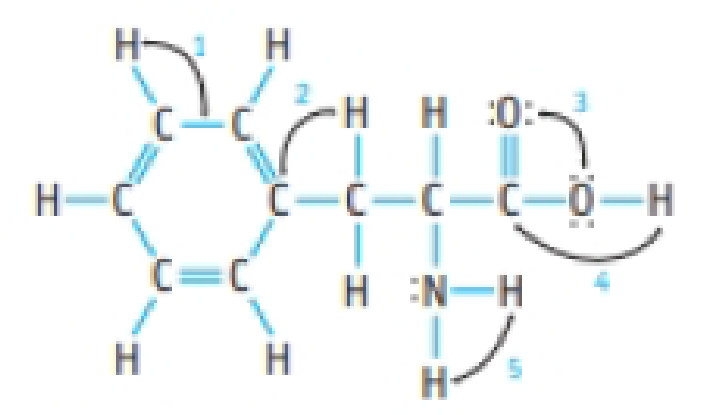Chapter 8, Problem 25PS

Chapter
Section
Textbook Problem

Phenylalanine is one of the natural amino acids and is a “breakdown” product of the artificial sweetener aspartame. Estimate the values of the indicated angles in the amino acid. Explain why the —CH2—CH(NH2)—CO2H chain is not linear.Interpretation Introduction

Interpretation: The indicated bond angles in the given molecule should be identified also why the -CH2-CH(NH2)-CO2H chain is not linear should be explained.

Concept Introduction:

Concept Introduction:

• Lewis structures are diagrams that represent the chemical bonding of covalently bonded molecules and coordination compounds.

VSEPR Theory:

As the name itself indicates that the basis for this theory is the electron pair that is bonded electron present in either single or double bonds or lone pair electrons, present in the valence shell tends to repel each other which then tend to be in position in order to minimize the repulsions. The steps involved in the theory in describing the geometry is as follows,

• The first step is to draw the correct Lewis structure for the molecule.
• Then, the electron domain around the central atom should be counted and the geometry that matches with that type of domain in VSEPR should be determined.
• Finally, the geometry is predicted by using the orientation of atoms.

The molecules with considering the domains of type AB2 will tend to have shape like linear or bent if the central atom have lone pair of electrons with it, type AB3 will have shape like trigonal planar, type AB4 will have shape like tetrahedral or square planar, type AB5 will have trigonal bipyramidal and AB6 will have shape like octahedral respectively.

Electron Domain: In VSEPR theory, both the lone pair and the bonded pair are together considered as electron domain regardless of the type of bond in which the bonded pair presents.

Bond angle: The angle formed between 2 bonds which the bonds originate from the same atom in a chemical compound.

Compounds with linear geometry contain central atom with no lone pairs and two other bonded atoms. The bond angle present in such compound is 180o.

Compounds with trigonal planar makes bond angle equal to 120o since three atoms gets bonded with the same central atom.

Explanation

Phenylanine is one of the natural aminoacid s and is a breakdown product o the artificial sweetener aspartame.

The value of the indicated angel in the amino acid is 1=1200,2=1090,3=12004

Still sussing out bartleby?

Check out a sample textbook solution.

See a sample solution

The Solution to Your Study Problems

Bartleby provides explanations to thousands of textbook problems written by our experts, many with advanced degrees!

Get Started

After about 50 years of age, bones begin to lose dens.

Nutrition: Concepts and Controversies - Standalone book (MindTap Course List)

What accounts for antibody diversity?

Introduction to General, Organic and Biochemistry

What is the difference between an isotope and an ion?

Horizons: Exploring the Universe (MindTap Course List)